# How To Calculate Your Solar Power Budget

Posted on 04/20/2018 at 12:00 by Daniel Stieler, PhdAs we learned in the last post, there are many considerations when calculating your solar power budget.

With that in mind, we will apply what we learned to an example application and show how to use the formulas discussed previously to size your solar and storage element to best fit your system.

We will focus on a powering an Engel MHD13F-DM freezer using solar to keep medical supplies frozen in a remote area in southern Africa.

According to the performance data listed for the freezer on the manufacturer’s website, if the system is operated in an ambient temperature of 25C with a setpoint temperature of -5C the freezer will draw power from a 12V system at a rate of 1.6Ahr/hour.

If the freezer is operated at an ambient temperature of 30C the current draw at 12V will be 2.5Ahr/hour. The system needs to operate 24 hours per day. The daily power draw is calculated using the following formula: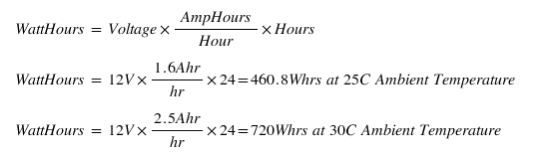Since the freezer system operates off of a 12V battery system, there will be no additional losses in converting the battery voltage to a voltage usable by the freezer. So for this case we do not need to worry about the power converter efficiency discussed in our last post.

For the charge controller we will choose a Galley Power GP2 series charge controller programmed to charge the AGM type lead acid battery. These are high performance charge controllers with a true maximum power point tracking algorithm implemented.

They will transfer the most power from your panel to a battery. The charge controllers track maximum power point with 99.7% efficiency and and have less than four percent loss in the power conversion from solar to battery making the overall conversion efficiency of the system about 96%. To account for the efficiency loss in the charge controller we will use the following formula: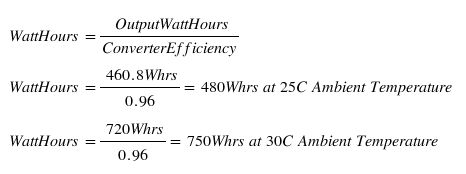So, the system will require 480Whrs to 750Whrs of energy produced by the solar panel each day depending on the ambient temperature.

Using the PVWatts calculator from NREL we find that there is data available in Zimbabwe, so that will be used for our estimate of solar illumination.

We will assume that the solar will be deployed flat on the ground making it easy to move the system from place to place and redeploy.

This means that in the PVWatts calculator the Tilt Angle must be set to 0 degrees.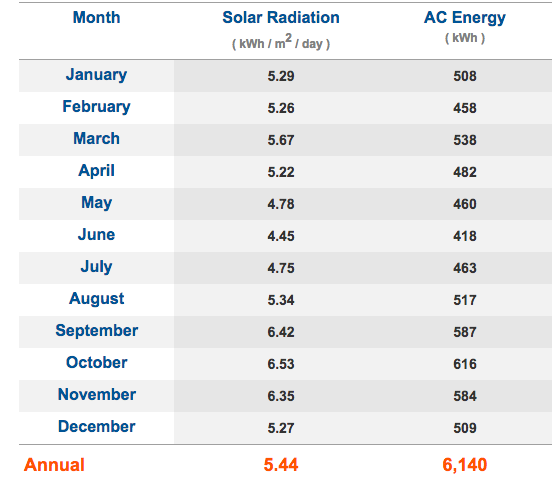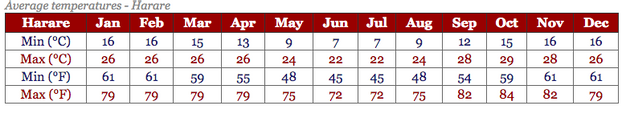Climate - Zimbabwe

From the report given by the PVWatts calculator we see that June is the month with the least solar radiation, with an average of 4.45kWh/m^2/day. At this time of year the maximum temperature is about 22C, with the minimum around 11C.

The month with the most solar radiation is October with an average solar radiation of 6.53kW/m^2/day. At this time of year the maximum temperature is about 29C and the minimum is 15C.

The units on illumination are kW/m^2/day and the standard illumination for solar panels is 1kW/m^2, so in this case a panel will receive an average of 4.45 hours of illumination per day in June and 6.53 hours in October.

The information from the freezer manufacturer was for running at a consistent 25C or 30C ambient temperature, so to optimize the system the actual draw will need to be quantified.

For this application we use a lead acid battery as the storage element since the freezer is specially design to pair with this type of battery.

The self-discharge rate of a lead-acid battery is 0.1-0.65% per day. If we want the system to have 2 days of reserve battery power, then the battery size can be calculated as follows: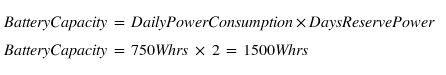We used the 750Whrs capacity in the calculation since that is the worst case. We are not relying on the battery to hold a charge for a long period of time and the self-discharge is so low it can be neglected.

To determine the battery capacity in amp-hours you just need to divide the Watt-hour capacity by 12V. So the minimum battery size would be 125Ahr at a 2.5A current draw.

The capacity is specified at a specific current draw since actual battery capacity will vary depending on the current draw. As the current draw increases battery capacity will decrease.

For example, a Trojan SCS225 specifies a 20-hour rate capacity of 130Ahr and a 5-hour rate discharge at 105Ahr. The discharge rate at each capacity can be calculated as follows: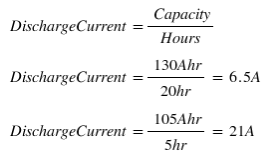Since the maximum current draw for this application is <3A, the battery capacity will be at least 130Ahr. A more accurate capacity could be determined through discharge testing of the battery, but we will use 130Ahr for our calculations.

Finally, we would like the battery to be able to fully charge in 2 days with the solar panel while still operating the freezer, so to calculate the solar panel size we will use the following formula: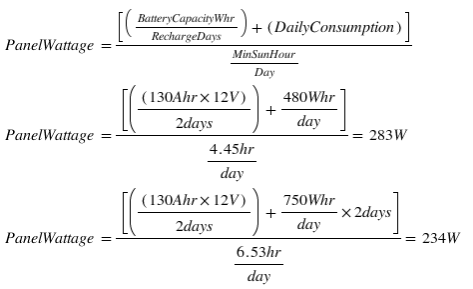This shows that the panel size would need to be at least 283W, with the winter conditions at the lower illumination and temperature the limiting situation.

In testing though this would probably not be true. The ambient temperature drops much lower for most of the day than the cooler operating temperature of 25C or 30C, and the current consumption vs ambient temperature drops quickly as well.

Testing is always important for system optimization. It is likely, based on the daily temperature cycle, that a PowerFilm standard 220W panel could easily support this freezer system.

This same kind of step by step logic and planning is crucial to secure a solid solution that will perform in real-world conditions.

Do you have need for a custom solution? Need help in determining what technology would be best for you? We can help.